## 5  Injectives and products in S

We know from Section A 6, and from the work on continuations by others cited there, that binary products are the crucial issue. These studies have also taught us that we must take just one step at a time. In Section 7 below we sketch the construction of products in S as coproducts of algebras, but the motivation of that approach involves the anticipation of results that ought to follow from the conclusion itself.

In this section we argue forwards from the structure in the given category S, using basic (albeit pedestrian) categorical ideas, and sticking to the objects rather than their algebras of predicates. The plan is to regard S-objects as equalisers in S as in Proposition 4.14, and then use the given products in S.

However, the universal property of these given products is only tested by diagrams of the form Γ→ A× B with Γ∈obS, so we need to generalise this to Γ∈obS. This is more difficult than the situation for colimits in Proposition 4.15: in this case we need to be able to turn maps {YE}→ A into YA, which is just the property of injectivity from which we began in Remark 2.1.

Definition 5.1 An object A of S is said to be injective if, for any subspace inclusion {YE}↣ Y and map f:{YE}→ A, there is some (not necessarily unique) map h:YA such that f=E;h.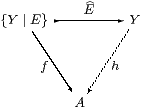Proposition 5.2 An object A of S is injective iff it is a retract of some ΣU (by which we still mean the exponential in S). Indeed, when AobS is injective, there is a map α:Σ2 AA such that ηA;α=id, though this need not satisfy the other equation that is required for (A,α) to be an Eilenberg–Moore algebra, namely µA;α=Σ2α;α.

Proof    (⇐) Suppose first that A ≡ ΣU, and put α ≡ ΣηU. By Definition 4.5, any morphism H:{YE}→ A in S satisfies

 H
=
 H
A;α  =
 E
YH;α  =
 E
;h,

where h ≡ ηYH;α is in S. The result extends to retracts of ΣU by composition with the inclusion and surjection.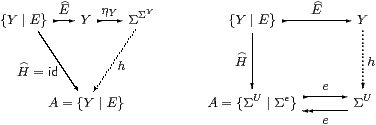(⇒) The composite {YE}↣Σ2 Y is a subspace by Corollary 4.13; injectivity says that this is split by h. When AYobS, α ≡ h satisfies ηA;α=id.         ▫

Corollary 5.3 S has enough injectives: each object {YE} is a subspace of some injective Σ2 Y.         ▫

Examples 5.4 In LKSp, the injectives are the continuous lattices equipped with the Scott topology [Sco72a], whilst the algebras are also distributive. In Set, injectives are (sets that carry the structure of) complete lattices, and algebras are powersets (which, classically, are complete atomic Boolean algebras).         ▫

Lemma 5.5 Any finite product of injectives (or algebras) is again injective (respectively, an algebra).

Proof    For the terminal object, α ≡ !ΣΣ.

For injectives (A,α) and (B,β), we define P0 ≡ Σ2π0;α:Σ2(A× B)→ A and P1 ≡ Σ2π1;β :Σ2(A× B)→ B, and then ηA× B;<P0,P1>=id. This follows from naturality of η, as illustrated in the right-hand trapezium below.

Moreover, if (A,α) and (B,β) are algebras then

 Σ2;;π0 = Σ4π0;Σ2α;α = Σ4π0;ΣηΣ A;α = ΣηΣ(A× B);Σ2π0;α = ΣηΣ(A× B);;π0

so (A× B,<P0,P1>) is also an algebra.         ▫

Lemma 5.6 The functor SS preserves the terminal object.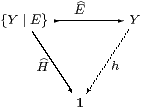Proof    Since 1 is injective, E;!Y is the only map H:{YE}→1.         ▫

Lemma 5.7 The functor SS preserves binary products of injectives.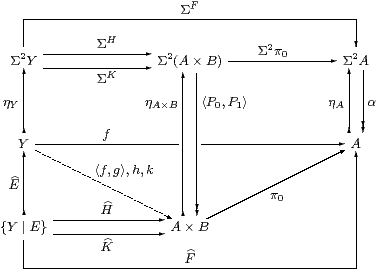Proof    Let F:{YE}→ A and G:{YE}→ B in S. Put

 f   ≡   ηY;ΣF;α     and     g   ≡   ηY;ΣG;β,

so F=E;f and G=E;g by Proposition 5.2, and <f,g> is given by the product in S.

Then HE;<f,g>:{YE}→ A× B satisfies H0=F and H1=G.

Suppose that H and K both satisfy these equations. Using Lemma 5.5, put

 h   ≡    ηY;ΣH;     and     k   ≡   ηY;ΣK;,

so H=E;h and K=E;k by Proposition 5.2.

Since ΣH2π0FK2π0, we have h0=f=k0 by the way in which h, k and f were constructed, and similarly h1=g=k1. Hence h=k=<f,g>, as these maps are in S, so H=K.         ▫

Now we can begin the main business of this section. In view of Corollary 5.3, we may assume that the S-objects of which we want to form the product are given by Σ-split pairs of maps between injectives. In fact this restriction will become redundant, as the argument below can be used first to show that all products are preserved, and then to construct products of non-injective pairs.

Lemma 5.8 Let (YZ)∈obS and WobS. Then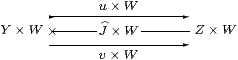is also an object of S. It is called (YZW because, at least when U, V and W are injective, this is the product in S. In the comprehension notation,

 EW
:{Y ∣ E}× W   ≡   {Y× W ∣ EW} ↣ Y× W.

This is functorial with respect to

g:W1→ W2,     where   {Y ∣ E}× g ≡
 Eg

(recall that id{YE}=E) and to

 F
:{Y1 ∣ E1}→{Y2 ∣ E2},     where
 F
× W ≡
 FW
.

Proof    Apply (−)W to the equations in Definition 4.2 to get JWu× W=id and

 Σv× W;JW;Σu× W  =   Σv× W;JW;Σv× W.

Proposition 4.14 said that the formal pairs are equalisers, which property commutes with products. In comprehension notation, Σv× W;JW=EW.

The formula for {YEg is merely naturality of E().

Using the injectivity of Y2, we may write F as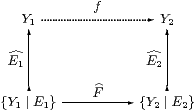for some (not necessarily unique) f, where (by Definition 4.5) F=F;E2=E1;f, so Ff;E1. Applying (−)× W to the commutative square, the formula for F× W has to be E1;(f× W)=G where G≡(Σf;E1)W=FW.         ▫

Remark 5.9 Alternatively, one could show directly that Eg and FW are morphisms, and that they satisfy the equations for the product functor. For FW, we need to use the strength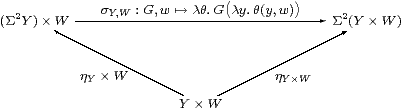for the monad, which is a natural transformation and obeys the equation shown.

Remark 5.10 The product of two Σ-split pairs must form a pullback (intersection) in S, in the same way that the product of any two maps in a category gives rise to a pullback.Unfortunately, our “comprehension” notation does not admit intersections — either abstractly or in the leading example of LKLoc — so we still have to find an E on Y1× Y2 to describe the subspace. If E1Y2 and E2Y1 commute (as they do if E1e1 or E2e2) then their composite encodes the intersection. But it turns out that, even if E1Y2;E2Y1E2Y1;E1Y2, either of them will do the job: of course products and pullbacks are unique up to isomorphism, but we have two representations of them.

Lemma 5.11 The compositeis an object of S, where

uu1× Y2;Z1× u2=Y1× u2;u1× Z2=u1× u2
vv1× Y2;Z1× v2=Y1× v2;v1× Z2=v1× v2

 J
Z1×
 J2
;
 J1
× Y2
 J1
× Z2;Y1×
 J2

 E
v;J  =  Y1×E2;E1× Y2  ⊂Y1× Y2  Y1×E2

the inclusion on the last line being in the sense of Notation 4.4.

Proof    Clearly u;J=id, whilst

 E
≡  v;
 J
=
v1× Y2Z1× v2Z1×
 J2
 J1
× Y2     definitions of v and J
=
v1× Y2Z1×
 E2
 J1
× Y2     definition of E2
=
Y1×
 E2
v1× Y2
 J1
× Y2     v1 central
=
Y1×
 E2
 E1
× Y2.     definition of E1.

Using the given equations of the form vi;Ji;ui=vi;Ji;vi, we must prove this equation for the new Σ-split pair:

v;
 J
;u
=
Y1×
 E2
;
 E1
× Y2;v1× Y2;Z1× v2
=
Y1×(v2;
 J2
;u2);(v1;
 J1
;u1)× Z2
=
v;
 J
;v,

using centrality of u2, and the same argument with v in place of u.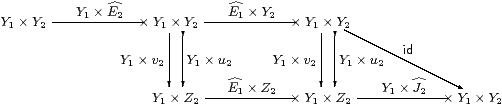Finally, Y1×E2;E1× Y2;Y1×E2  =  Y1×E2;E1× Y2 by centrality of u2 and v2 with respect to E1, and u2;J2=id.         ▫

Proposition 5.12 The category S has finite products, where

 {Y1 ∣ E1}×{Y2 ∣ E2}   =  {Y1× Y2 ∣ E1Y2;E2Y1},

whilst the product of the morphisms F1:X1X1 and F2:X2X2 is F1Y2;F2Y1.

Moreover, the functor SS takes products in S to products in S.

Proof    First consider the product of two objects X1,X2obS, expressed in S by means of their standard resolutions, so the products Σ2 X1×Σ2 X2 etc. are preserved (Lemma 5.7). The formal product of the Σ-split pairs (Lemma 5.11) provides an equaliser in S (Proposition 4.14), which is the required product, isomorphic to X1× X2, i.e. this is preserved.

The construction is then applicable to any two Σ-split pairs, so all binary products exist in S. Functoriality follows from that in Lemma 5.8.         ▫

Remark 5.13 The abstract situation of idempotents є ≡ (E2)Y1 and є′ ≡ (E1)Y2 (in this case on the object ΣY1× Y2) such that є;є′ and є′;є are also idempotent is studied in Lemma D 7.1. It is shown there that the splittings of є;є′ and є′;є are isomorphic and form a pushout diagram, in our case of the algebras ΣX1× X2 etc. corresponding to objects in the above pullback.